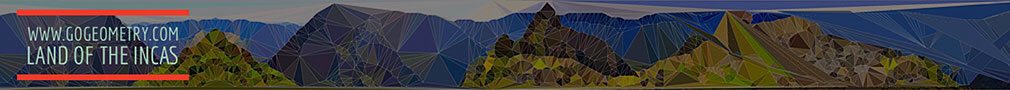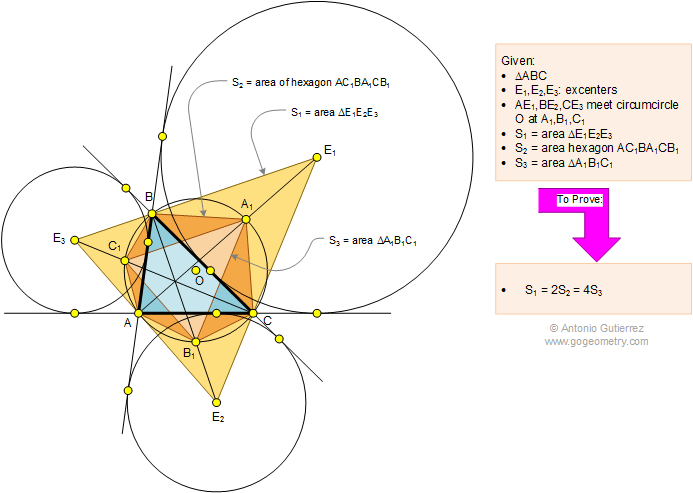# Math: Geometry Problem 1112: Triangle, Excenters, Circumcircle, Circle, Hexagon, Area. Level: High School, College

< PREVIOUS PROBLEM  |  NEXT PROBLEM >

 The figure below shows a triangle ABC with the excenters E1, E2, and E3. Lines AE1, BE2, and CE3 meet circumcircle O at A1, B1, and C1, respectively. If S1, S2, and S3 are the areas of triangle E1E2E3, hexagon AC1BA1CC1, and triangle A1B1C1, respectively, prove that S1 = 2.S2 = 4.S3.Home | Geometry | Problems | All Problems | Open Problems | Visual Index | 1111-1120 | Triangles | Hexagon | Area | Triangle area | Excenter | Circumcircle | Email | Post a comment or solution
Last updated: Apr 24, 2015 by Antonio Gutierrez